# Static Variables in C++

## Static Variables in C++ with Examples:

In this article, I am going to discuss Static Variables in C++ Language with examples. Please read our previous article, where we discussed Local and Global Variables in C++ with examples.

##### Static Variables in C++:

We have already covered local and global variable so with the help of that we will explain the static variables.

int v = 0;
void fun(){
int a = 9;
v++;
cout << a << ” ” << v << endl;
}
int main(){
fun();
fun();
fun();
}

Here is an example. In this example, we have one global variable that is ‘v’ which is initialized with the value 0, then there is a function ‘fun’ which is having a local variable ‘a’ which is initialized with the 9. Inside the ‘fun()’ function, we have incremented ‘v’ as ‘v++’ and then display the value of ‘a’ and ‘v’. Then from the main function, we have called the function ‘fun()’ 3 times. So the main is not having anything just it is calling function ‘fun’ function 3 times.

##### How does it work?

Now let us see how it works. Main and ‘fun’ are loaded inside the code section then also variable ‘v’ is created inside the code section. We know well that global variables are created inside the code section at loading time before the execution of a program.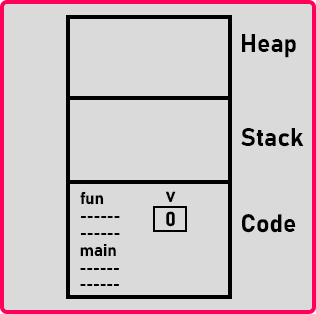Now main function start. So, there is nothing inside the main function so a dummy activation record we are taking, there is no variable, so no memory at all. Then the function ‘fun’ is called and the ‘fun’ activation record is created with a variable a with a value of 5.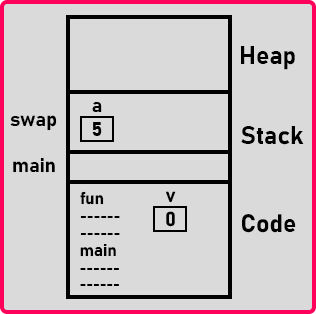Next ‘v++’ then ‘v’ becomes one. Then print ‘a’ and ‘v’, ‘a’ is 5 and ‘v’ is 1.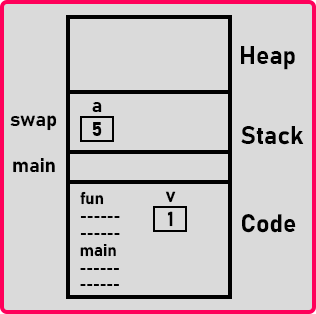So ‘a’ is 5 and ‘v’ is 1 in the first call of function ‘fun’ inside the main function. Once the function ends this activation record is deleted then it comes back to the main function, and what is the next line? Again, the function call. So again, the activation record is created, and ‘a’ is created with a value of 5. Then ‘v++’, ‘v’ becomes 2, and then print ‘a’ and ‘v’. Now ‘a’ is 5 and ‘v’ is 2. For a better understanding, please have a look at the below image.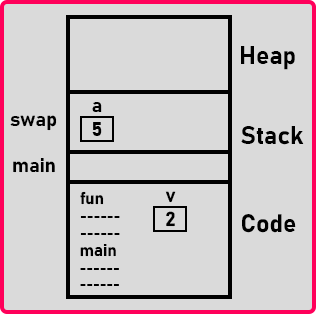Now once the function ends, again the activation record is deleted. Again, we have called the function ‘fun’ so the same steps will repeat. Finally, we got the values of ‘a’ and ‘v’ are 5 and 3.

##### What are Static Variables in C++?

Now let us understand static variables. Here, ‘a’ is a local variable for function fun, it is created and destroyed whenever the function is called. ‘v’ is a global variable which is remaining there throughout the program inside the code section and both functions can access it. But here in our example, only function ‘fun’ is accessing ‘v’, main is not accessing, there is no problem. It’s not mandatory that every function should access it.

We want the ‘v’ variable inside the function ‘fun’. If we bring that variable inside ‘fun’ then it’s not global, it became local. So, there are two points about global – it can be accessible everywhere and it will remain always in the memory.

We want it to remain always in the memory and we don’t want it to be accessible by all the functions. We want it to be accessible only by function ‘fun’. So, we will write static. So, if we want to make variable ‘v’ for accessible only by function ‘fun’ and also, we want it to remain always in the memory then we can write it as,

void fun(){
static int v = 0;
int a = 9;
v++;
cout << a << ” ” << v << endl;
}

So Static Variables in C++ are the variables that remains always in the memory. They are just like a global Variable. Only the difference between global and static variables is global variables can be accessed in any function and static variables are accessible only inside the function in which they are declared.

A static variable is not created every time we call a function. They are just created only once which is at the loading time. Now let us see the program for static variables.

##### Static Variables Program in C++:
```#include <iostream>
using namespace std;
void fun()
{
static int v = 0;
int a = 10;
v++;
cout << a << " " << v << endl;
}

int main()
{
fun();
fun();
fun();
}
```
##### Output: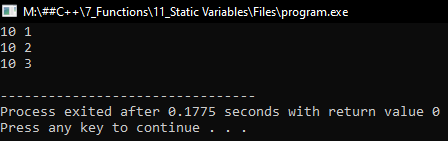##### Static variables Key Points
• They have a local scope but remain in memory throughout the execution of the program
• They are created in the code section
• They are history-sensitive
##### When to use Static Variable in C++?
• We should use a static variable whenever we want to reuse the modified value of the variable inside a function in the next function call.
• When we want all the objects to maintain a single copy of the class variable.
##### Advantages of C++ static keyword

Memory efficiency: Now we don’t need to create an instance for accessing the static members, so it saves memory. Moreover, it belongs to the type, so it will not get memory each time when the instance is created.